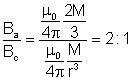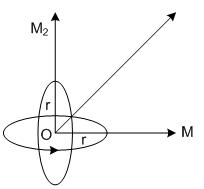×#### Thank you for registering.

One of our academic counsellors will contact you within 1 working day.

Click to Chat

1800-1023-196

+91-120-4616500

CART 0

• 0

MY CART (5)

Use Coupon: CART20 and get 20% off on all online Study Material

ITEM
DETAILS
MRP
DISCOUNT
FINAL PRICE
Total Price: Rs.

There are no items in this cart.
Continue Shopping• Complete Physics Course - Class 11
• OFFERED PRICE: Rs. 2,968
• View Details

```Assignment and Solutions for Test

Q1. The area of hysteresis loop of a material is equivalent to 250 Joule. When 10 kg material is magnetised by an alternating field of 50Hz then energy lost in one hour will be if the density of material is 7.5 gm/cm3.

(A)       6×104 Joule                                       (B)       6×104 Erg

(C)       3×102 Joule                                       (D)       3×102 Erg.

Solution: (A) E = nA Vt = nA m/d t

= 50 × 250 × 10 × 3600 / 7.5 × 103 = 6 × 104 Joule

Q2. The corecivity of a bar magnet is 100 A/m. It is to be demagnetised by placing it inside a solenoid of length 100 cm and number of turns 50. The current flowing the solenoid will be-

(A)       4A                   (B)       2A                   (C)       1A                   (D)       zero.

Solution:  (B) H = ni          i = H / H = 100/50 = 2A

Q3. A current i is flowing in a conductor of length l. When it is bent in the form of a loop then its magnetic moment will be-

(A) l2i / 4π                        (B)       l2 / 4π                  (C)       4π / l2i                   (D)       4pl2i.

Solution: (A) M = iA = i π r2     But 2πr = l      M = iπ l2 / 4π2 = il2 / 4π

Q4. A rod of ferromagnetic material with dimensions 10 cm×0.5 cm×0.2 cm is placed in a magnetic field of strength 0.5×104 amp/m as a result of which a magnetic moment of 5 amp-m2 is produced in the rod. The value of magnetic induction will be-

(A)       0.54 Tesla                                          (B)       0.358 Tesla

(C)       2.519 Tesla                                       (D)       6.28 Tesla.

Solution:  (D) B = μ0 (l + H) = μ0 (M/V + H)

4 × 3.14 × 10–7 × [5 / 10–6 + 5000] = 6.28 Tesla.

Q5. The ratio of intensities of magnetic field in the axial and equatorial positions of a magnet will be-

(A)       1 : 4                 (B)       4 : 1                 (C)       1 : 2                 (D)       2 : 1

Solution:        (D)Q6. The resultant magnetic moment due to two current (i) carrying concentric coils of radius r, mutually perpendicular to each other will be-

(A)       √2iπr2             (B)       √2ir2              (C)       √2ir                (D)       √2πr2

Solution: (A) M' = √2M = √2πr2ir

Q7. The magnetic susceptibility of a paramagnetic material at –73oC is 0.0075 then its value at –173oC will be-

(A)       0.0045            (B)       0.0030            (C)       0.015              (D)       0.0075.

Solution: (C) For paramagnetic materials X ∝ 1/T

∴ C2/X1 = T1/T2 or X2 = 0.0075 × 200 / 100 = 0.015

Q8. The area of cross-section of three magnets of same length are A, 2A and 6A respectively. The ratio of their magnetic moments will be-

(A)       6 : 2 : 1           (B)       1 : 2 : 6           (C)       1 : 4 : 36         (D)       36 : 4 : 1

Solution:  (B) M μ m μ A (Area of cross-section)

M1 : M2 : M3 = 1 : 2 : 6

Q9. If the radius of a circular coil is doubled and the current flowing in it is halved then the new magnetic moment will be if its initial magnetic moment is 4 units-

(A)       8 units            (B)       4 units            (C)       2 units            (D)       zero.

Solution: (A) M = π R2 Ni

Q10. Newton/Weber is the unit of-

(A)       H                    (B)       M                    (C)       B                    (D)       Φ

Solution:        (A) F = Bil = Φ/A il or F/Φ = i/l = H

Q11. The inner and the outer radii of a toroid are 9cm and 11cm respectively and the number of turns in it is 3140. A magnetic field of 2.5 Tesla is produced in it when a current of 0.5 ampere is passed in it. The permeability of core material is (in Henry/meter)-

(A)       10–1                 (B)       10–2                 (C)       10–3                 (D)       10–4

Solution: (C) m = B/H = B / Ni/πr = 2.5 × 2 × 3.14 × 0.1 / 3140 × 0.5 = 10–3 Henry / meter

Q12. In the above problem the relative permeability of the core will be-

(A)       684.5              (B)       864.7              (C)       369.4              (D)       796.2

Solution: (D) m1 = m / m0 = 10–3 / 12.57 × 10–7 = 796.2

Q13.  A magnetising field of 5000 A/m produces a magnetic flux of 5×10–5 Weber in an iron rod. If the area of cross-section of the rod is 0.5 cm2, then the permeability of the rod will be (in henry/m)-

(A)       1 × 10–3             (B)       2 × 10–4          (C)       3 × 10–5          (D)       4 × 10–6

Solution: (B) B = Φ/A = nH     m / Φ/AH = 5×10–5 / 0.5 × 10–4 × 5 × 103 = 2 × 10–4 Henry / m

Q14. In the above problem the magnetic susceptibility of the rod will be-

(A)       158.2              (B)       198.0              (C)       295.3              (D)       343.6

Solution:  (A) m1 = m / m0 = 1 + X        X = m / m0 – 1 = 2 × 10–4 / 12.56 × 10–7 – 1 = 159.2 – 1 = 158.2
```### Course Features

• 101 Video Lectures
• Revision Notes
• Previous Year Papers
• Mind Map
• Study Planner
• NCERT Solutions
• Discussion Forum
• Test paper with Video Solution